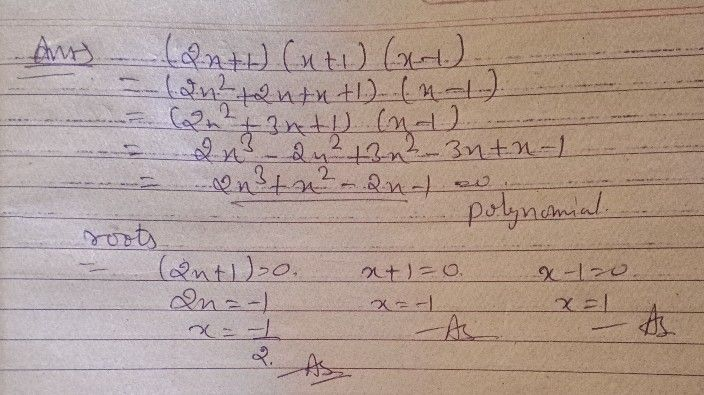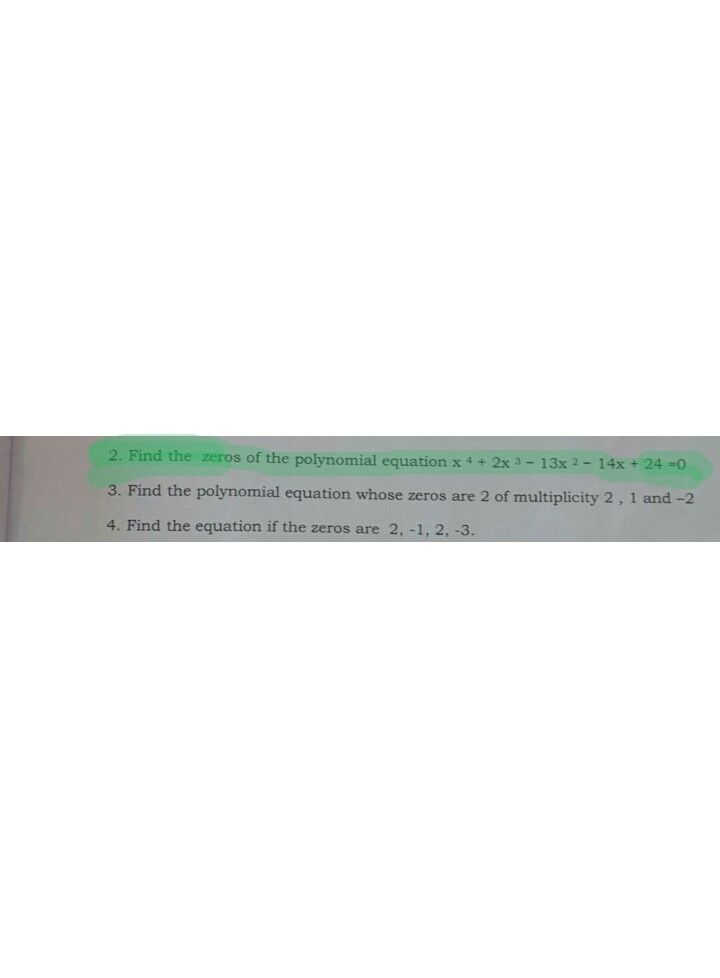Symbol
Problem$A$ Perform as instructed $1.$ Find the roots of $\left(2x+1\right)\left(x+1\right)\left(x-1\right)$ $=0$ $2.$ Find the $2$ zeros of the polynomial equation $x^{4+2x^{3-13x^{2-}}}$ $14x+24=0$ $3$ Find the polynomial equation whose zeros are $2$ of multiplicity $2,$ $1$ and $-2$ $4$ Find the equation if the zeros are $2,-1,$ $2$ $-3.$
10th-13th grade
Algebra
Search count: 112
SolutionQanda teacher - Nitesh77ok
pls ask if there is any doubt regarding the answer
thank youStudent
thankyou sirsir can you answer this one too?